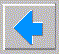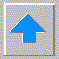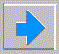# Lecture 3 - Intelligent Agents and blind search

• Agent (RN s3.1)
• exists in an environment
• inputs are sensor perceptions (of environment)
• output are actions (on environment)
• may be (non)deterministic, may have memory, may be conscious
• Rational agents strive to maximize performance
• simplest rational agent - lookup table
• lookup tables explode fast, are not autonomous, hard to hand craft, not conscious?
• What are environments like?
• accessible?
• deterministic?
• discrete time?
• episodic?
• static?
• How do agents work?
• ```   loop:
sense environment (input)
update internal state
decide on action
perform action (output)
```
• Use environment simulator to develop and test agents
• ```   initialize
loop:
update environment (outside influences)
for each agent
sense; update state; decision; action;
done?
```
• Types of agents
• Reflex agent: no internal state (memory)
• input; decide; act (e.g. LUT);
• Goal based agent
• add goal test
• Utility based agent
• goal and cost function (try for best goal state)
• cost function may be goal state cost or whole path cost (e.g. additive)
• Constraint satisfaction problems
• Problem = initial state, operator (set of successor states), goal test, cost function
• Solution = sequence of states from initial state to goal state
• Examples
• Puzzles: 8 queens, 15 problem, Rubic's cube, missionaries+cannibals, cryptarithmetic
• Games: chess, checkers, sheshbesh, number game, tictactoe
• NP complete problems: Travelling Salesman Problem (TSP), map coloring, etc.
• Robotic problems: motion, navigation, ALIFE
• Industrial problems: VLSI placing, routing, division
• Misc. AI: theorem provers, expert systems
• Search problems
• Difference between search and planning
• Review of Graph Theory
• digraph, tree, node, root, leaf, depth, fringe
• expanding a state means generating all daughter states
• Relation between searching and trees
• forward chaining vs. backwards chaining
• dead-ends and backtracking (Prolog)
• Basic search procedure
• ```   initialize;
loop:
if no expandable leaf - fail;
choose a leaf;
goal test:
if succeed
then return solution
else expand leaf
```
• To choose means employing a strategy
• completeness
• optimality
• time complexity
• memory complexity
• Blind (uninformed) search
• depth first
• depth limited
• iterative deepening
• bidirectional (if invertible)
• Informed (heuristic) search with state cost (or constraint satisfaction)
• gradient descent (GD)
• GD with restarts
• GD with pocket
• Downhill Simplex
• Simulated annealing
• Informed search with path cost (assume only have real cost after expansion)
• Heuristics
• how to find
• admissable (less cost than real)
• best first - greedy heuristics (neither complete nor optimal)
• A* and variants